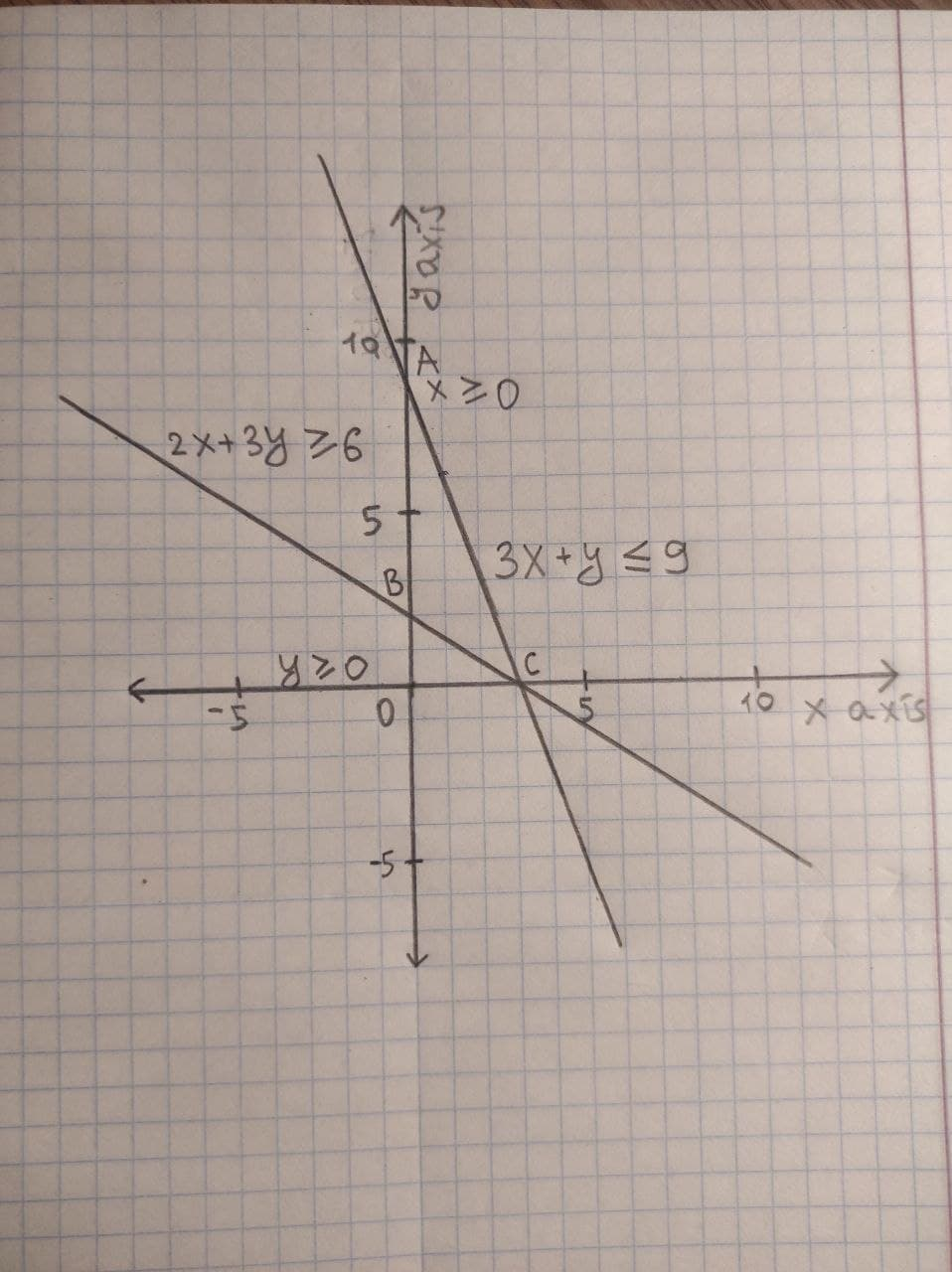# Graph the solution set of system of inequalities: x >= 0, y >= 0, 3x + y <= 9, 2x + 3y >= 6Lewis Harvey 2020-12-05 Answered
Graph the solution set of system of inequalities:
$x\ge 0,y\ge 0,3x+y\le 9,2x+3y\ge 6$
You can still ask an expert for help

## Want to know more about Inequalities systems and graphs?

• Questions are typically answered in as fast as 30 minutes

Solve your problem for the price of one coffee

• Math expert for every subject
• Pay only if we can solve itcheekabooy
The graph of system of inequalities is given by: